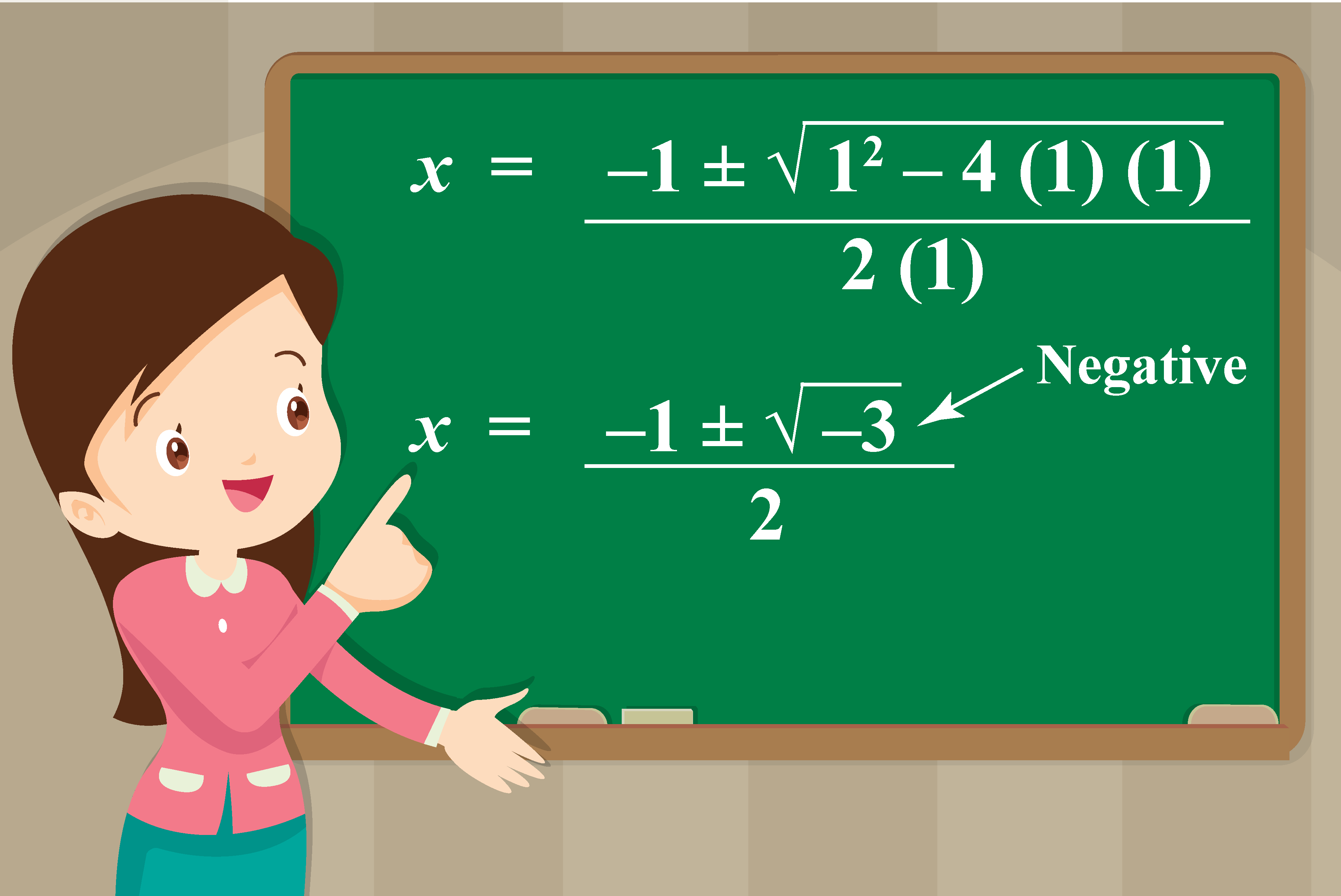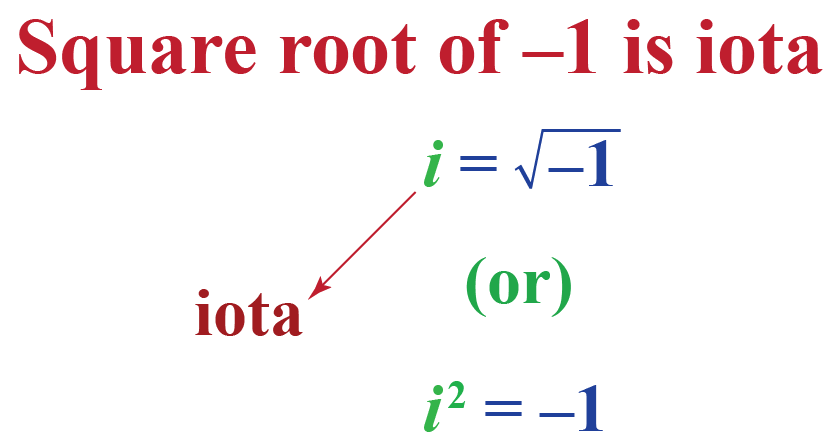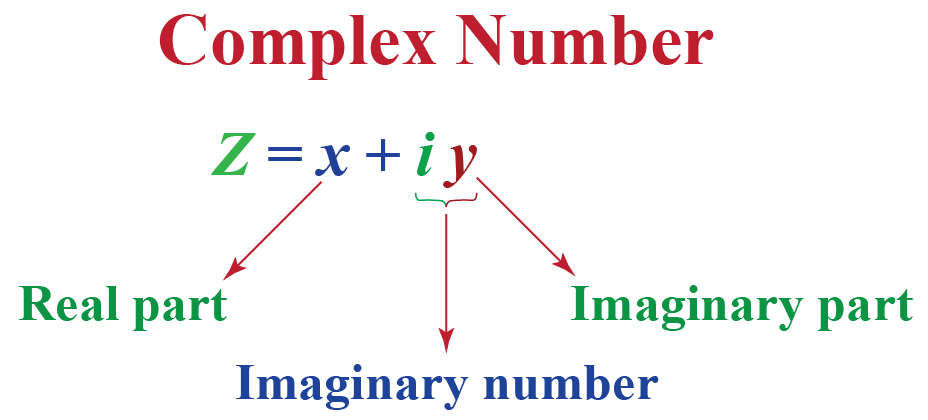# Complex Numbers

Complex Numbers
Go back to  'Numbers'

In this mini-lesson, we will explore the definition and the meaning of complex numbers and imaginary numbers.

While doing this, sometimes, the value inside the square root may be negative.

For example, while solving a quadratic equation $$x^2+x+1=0$$ using the quadratic formula, we get:So far we know that the square roots of negative numbers are NOT real numbers.

Then what type of numbers are they?

They are complex numbers. Let's learn in detail about complex numbers.

## What Are Complex Numbers?

### Square Root of Negative 1

The square root of negative 1 is "iota" and its value is $$i = \sqrt{-1}$$.The square of any real number is always positive. So "iota" is introduced to simplify the square roots of negative numbers.

### What Is an Imaginary Number?

An imaginary number is a number that results in a negative number when it is squared.

Let's try squaring some real numbers:

\begin{align} (-2)^2 &= -2 \times -2 =4\\[0.2cm] 5^2 &= 5 \times 5 =25\\[0.2cm] (1.3)^2&= 1.3 \times 1.3 =1.69 \end{align}

Is any of the result a negative number?

No!!

It means the square of any real number is always positive.

Then what number results in a negative square? It is an imaginary number.

An imaginary number is the product of a real number and iota $$(i)$$.

Here are some examples of imaginary numbers:

\begin{align} 3i &= 3 \sqrt{-1}= \sqrt{9} \times \sqrt{-1}= \sqrt{-9}\\[0.2cm] \sqrt{3}i &= \sqrt{3} \times \sqrt{-1}= \sqrt{-3} \end{align}

So in general:

 $$\sqrt{-x} = i \sqrt{x}$$Tips and Tricks
1. When the minus sign comes out of the square root, it becomes $$i$$.
2. $$i$$ is used to simplify $$\sqrt[n]{x}$$ where $$n$$ is an even number and $$x$$ is a negative number.

### What Are Complex Numbers?

A complex number is the sum of a real number and an imaginary number.

i.e., a complex number is of the form $$x+iy$$ and is usually represented by $$z$$.

Here both $$x$$ and $$y$$ are real numbers.

• $$x$$ is called the real part which is denoted by $$Re(z)$$.
• $$y$$ is called the imaginary part $$Im(z)$$.
• $$iy$$ is an imaginary number.Here are some examples of complex numbers:

$$2+3i, -2-5i, \,\,\dfrac 1 2 + i\dfrac 3 2$$, etc.

Every complex number indicates a point in the XY-plane. For example:

Complex Number

Corresponding Point
2 + 3i (2, 3)
-1 - 5i (-1, -5)
3 - 2i (3, -2)

You can see this in the following illustration. Here, you can drag the point by which the complex number and the corresponding point are changed.

## Operations on Complex Numbers

• The sum (or difference) of two complex numbers is a complex number whose real part and the imaginary part are obtained by just adding (or subtracting) the corresponding real parts and imaginary parts respectively. i.e.,
$$(a+ib)\pm(x+iy)$$= $$(a\pm x)+i(b\pm y)$$
• The product of two complex numbers is found by multiplying them considering as binomials. i.e.,
\begin{align} (a+ib)(x+iy)= ax+iay+ibx+i^2by\\[0.2cm] =ax+i(ay+bx)-by\,[\because i^2=-1]\\[0.2cm] = (ax-by)+ i (ay+bx) \end{align}
• To divide two complex numbers, we rationalize the denominator by multiplying and dividing by the conjugate of the complex number. \begin{align} &\dfrac{a+ib}{x+iy}\\[0.2cm] &=\dfrac{a+ib}{x+iy} \cdot \dfrac{x-iy}{x-iy}\\[0.2cm] &= \dfrac{ax-aiy+ibx+by}{x^2+y^2}\,\,\,\, [\because i^2=-1]\\[0.2cm] &= \dfrac{(ax+by)+i(bx-ay)}{x^2+y^2} \end{align}

## Solved Examples

 Example 1

Can we help Sophia express the roots of the quadratic equation $$x^2+x+1=0$$ as complex numbers?

Solution

Comparing the given equation with $$ax^2+bx+c=0$$, $a=1\\[0.2cm]b=1\\[0.2cm]c=1$

(This equation is as same as the one we saw in the beginning of this page).

Substitute these values in the quadratic formula:

\begin{align} x&= \dfrac{-b \pm \sqrt{b^2-4ac}}{2a}\\[0.2cm] &=\frac{-1 \pm \sqrt{1^{2}-4(1)(1)}}{2(1)} \\[0.2cm] &=\frac{-1 \pm \sqrt{-3}}{2}\\[0.2cm] \text{Here, } &\sqrt{-3} = \sqrt{-1} \times \sqrt{3} = i \sqrt{3}\\[0.2cm] x&= \frac{-1 \pm i\sqrt{3}}{2}\\[0.2cm] &= \frac{-1}{2}+ i\frac{\sqrt{3}}{2};\,\,\, \frac{-1}{2}- i\frac{\sqrt{3}}{2} \end{align}

Thus the roots of the given quadratic equation are:

 $$\frac{-1}{2}+ i\frac{\sqrt{3}}{2};\,\,\, \frac{-1}{2}- i\frac{\sqrt{3}}{2}$$
 Example 2

Express the sum, difference, product, and quotient of the following complex numbers as a complex number.

\begin{align} z_1&=-2+i\\[0.2cm]z_2&= 1-2i \end{align}

Solution

Sum:

\begin{align} z_1+z_2&= (-2+i)+(1-2i)\\[0.2cm] &=(-2+1)+ (i-2i)\\[0.2cm] &= -1-i \end{align}

Difference:

\begin{align} z_1-z_2&= (-2+i)-(1-2i)\\[0.2cm] &=(-2-1)+ (i+2i)\\[0.2cm] &= -3+3i \end{align}

Product:

\begin{align} z_1\cdot z_2&= (-2+i)(1-2i)\\[0.2cm] &=-2+4i+i-2i^2\\[0.2cm] &=-2+4i+i+2 \,\,\, [\because i^2=-1]\\[0.2cm] &=5i \end{align}

Quotient:

\begin{align} \dfrac{z_1}{z_2}&= \dfrac{-2+i}{1-2i}\\[0.2cm] &= \dfrac{-2+i}{1-2i} \cdot \dfrac{1+2i}{1+2i}\\[0.2cm] &= \dfrac{-2-4i+i+2i^2}{1-4i^2}\\[0.2cm] &= \dfrac{-2-4i+i-2}{1+4}\,\,\, [\because i^2=-1]\\[0.2cm] &= \dfrac{-4-3i}{5}\\[0.2cm] &=- \dfrac{4}{5}- i \dfrac{3}{5}\end{align}

Therefore,

 \begin{align} \text{Sum: }& -1-i\\[0.2cm] \text{Difference: } &-3+3i\\[0.2cm] \text{Product: } &5i\\[0.2cm] \text{Quotient: } &- \dfrac{4}{5}- i \dfrac{3}{5} \end{align}

## Interactive Questions

Here are a few activities for you to practice.

## Let's Summarize

The mini-lesson targeted the fascinating concept of Complex Numbers. The math journey around Complex Numbers starts with what a student already knows, and goes on to creatively crafting a fresh concept in the young minds. Done in a way that not only it is relatable and easy to grasp, but also will stay with them forever. Here lies the magic with Cuemath.

At Cuemath, our team of math experts is dedicated to making learning fun for our favorite readers, the students!

Through an interactive and engaging learning-teaching-learning approach, the teachers explore all angles of a topic.

Be it worksheets, online classes, doubt sessions, or any other form of relation, it’s the logical thinking and smart learning approach that we, at Cuemath, believe in.

## 1. What is an imaginary number?

An imaginary number is a number that results in a negative number when it is squared. It is the product of a real number and iota $$(i)$$.

## 2. What is square root of 1?

The square root of 1 is $$\pm 1$$.

But the square root of negative 1 is $$i$$.

Complex Numbers
grade 10 | Questions Set 1
Complex Numbers
Complex Numbers